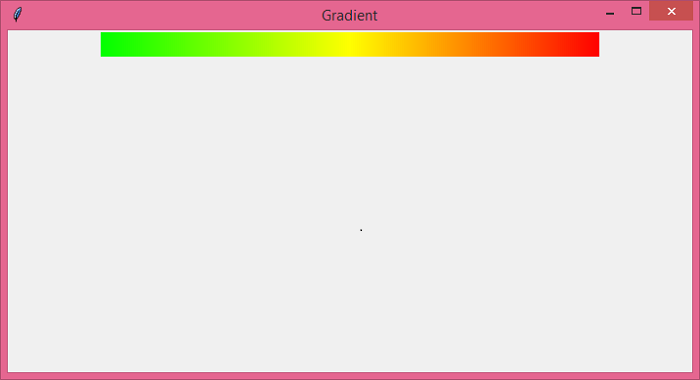# How to draw a scale that ranges from red to green in Tkinter?

A color gradient defines the range of position-dependent colors. To be more specific, if you want to create a rectangular scale in an application that contains some color ranges in it (gradient), then we can follow these steps −

• Create a rectangle with a canvas widget and define its width and height.

• Define a function to fill the color in the range. To fill the color, we can use hex values inside a tuple.

• Iterate over the range of the color and fill the rectangle with it.

## Example

# Import the required libraries
from tkinter import *
from tkinter import ttk

# Create an instance of tkinter frame
win = Tk()

# Set the size of the window
win.geometry("700x350")

# Define a function for filling the rectangle with random colors
def rgb(r, g, b):
return "#%s%s%s" % tuple([hex(c)[2:].rjust(2, "0")
for c in (r, g, b)])

gradient = Canvas(win, width=255 * 2, height=25)

# Iterate through the color and fill the rectangle with colors(r,g,0)
for x in range(0, 256):
r = x * 2 if x < 128 else 255
g = 255 if x < 128 else 255 - (x - 128) * 2
gradient.create_rectangle(x * 2, 0, x * 2 + 2, 50, fill=rgb(r, g, 0), outline=rgb(r, g, 0))

win.mainloop()

## Output

Running the above code will display a scale gradient that has some range of colors defined in it.